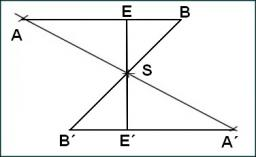# Circumference 6312

The triangle has a circumference of 35 cm. The first side is four centimeters larger than the second and, at the same time, 1 cm larger than the third side. Determine the sides of the triangle.

a =  13.3333 cm
b =  9.3333 cm
c =  12.3333 cm

### Step-by-step explanation:Did you find an error or inaccuracy? Feel free to write us. Thank you!

Tips for related online calculators
Do you have a linear equation or system of equations and looking for its solution? Or do you have a quadratic equation?